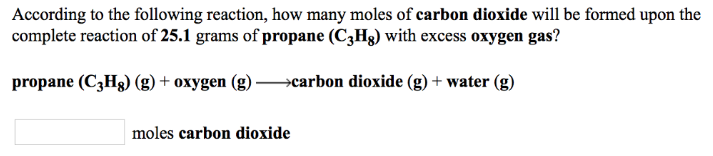Chemistry Stoichiometry Solution: According to the following reaction, how many mole...

# Solution: According to the following reaction, how many moles of carbon dioxide will be formed upon the complete reaction of 25.1 grams of propane (C3H8) with excess oxygen gas? propane (C3H8) (g) + oxygen (g) → carbon dioxide (g) + water

Problem

According to the following reaction, how many moles of carbon dioxide will be formed upon the complete reaction of 25.1 grams of propane (C3H8) with excess oxygen gas?

propane (C3H8) (g) + oxygen (g) → carbon dioxide (g) + waterView Complete Written Solution